## Cassini OvalsThe curves, also called Cassini Ellipses, described by a point such that the product of its distances from two fixed points a distanceapart is a constant. The shape of the curve depends on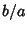. If, the curve is a single loop with an Oval (left figure above) or dog bone (second figure) shape. The caseproduces a Lemniscate (third figure). If, then the curve consists of two loops (right figure). The curve was first investigated by Cassiniin 1680 when he was studying the relative motions of the Earthand the Sun.Cassini believed that the Sun traveled around the Earth on one of these ovals, with the Earth at one Focus of the oval.

Cassini ovals are Anallagmatic Curves. The Cassini ovals are defined in two-center Bipolar Coordinates by the equation(1)

with the origin at a Focus. Even more incredible curves are produced by the locus of a point the product of whose distances from 3 or more fixed points is a constant.

The Cassini ovals have the Cartesian equation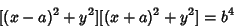(2)

or the equivalent form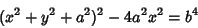(3)

and the polar equation(4)

Solving for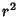using the Quadratic Equation gives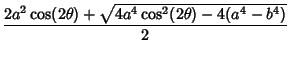(5)

If, the curve has Area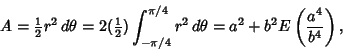(6)

where the integral has been done over half the curve and then multiplied by two andis the complete Elliptic Integral of the Second Kind. If, the curve becomes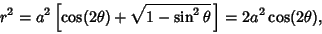(7)

which is a Lemniscate having Area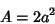(8)

(two loops of a curvethe linear scale of the usual lemniscate, which has area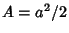for each loop). If, the curve becomes two disjoint ovals with equations(9)

whereand(10)

References

Gray, A. Cassinian Ovals.'' §4.2 in Modern Differential Geometry of Curves and Surfaces. Boca Raton, FL: CRC Press, pp. 63-65, 1993.

Lawrence, J. D. A Catalog of Special Plane Curves. New York: Dover, pp. 153-155, 1972.

Lee, X. Cassinian Oval.'' http://www.best.com/~xah/SpecialPlaneCurves_dir/CassinianOval_dir/cassinianOval.html

Lockwood, E. H. A Book of Curves. Cambridge, England: Cambridge University Press, pp. 187-188, 1967.

MacTutor History of Mathematics Archive. Cassinian Ovals.'' http://www-groups.dcs.st-and.ac.uk/~history/Curves/Cassinian.html.

Yates, R. C. Cassinian Curves.'' A Handbook on Curves and Their Properties. Ann Arbor, MI: J. W. Edwards, pp. 8-11, 1952.